# SSAT Middle Level Math : How to divide fractions

## Example Questions

### Example Question #11 : Fractions

Evaluate: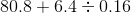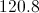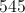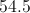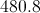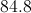Explanation:

By order of operations, divide first. Move the decimal point right two places in both dividend and divisor to make the divisor a whole number, and divide the resulting whole numbers: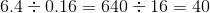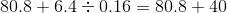Append a decimal point and a zero to 40, align the decimal points, and add: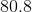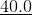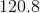### Example Question #12 : Fractions

Evaluate: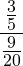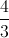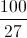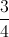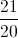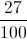Explanation:

Rewrite this horizontally as a division expression, rewrite as a product, cross-cancel, and multply across: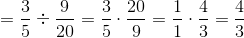### Example Question #13 : Fractions

Evaluate: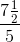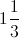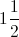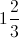Explanation:

Rewrite this horizontally as a division expression, rewrite as a product, cross-cancel, and multply across: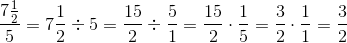Since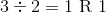,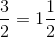### Example Question #14 : Fractions

Evaluate: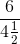Explanation:

Rewrite this horizontally as a division expression, rewrite as a product, cross-cancel, and finally multiply across: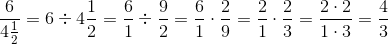Since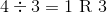,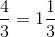,

### Example Question #11 : How To Divide Fractions

Which of the following yields the largest number?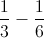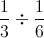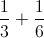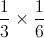Explanation:

When dividing by a fraction between 0 and 1, the answer will always get larger. In this case it is larger than simply adding the two fractions together.

### Example Question #16 : Fractions

Divide: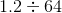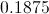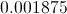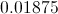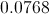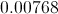Explanation:

Simply use the long division process. The quotient will be: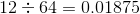### Example Question #17 : Fractions

Simplify: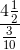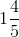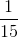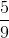Explanation:

Rewrite as a division and evaluate: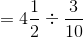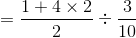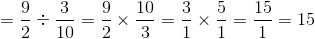### Example Question #18 : Fractions

Simplify: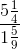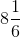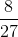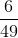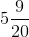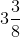Explanation:

Rewrite as a division, then solve: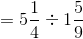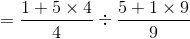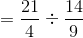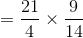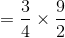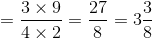### Example Question #19 : Fractions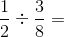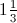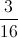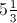Explanation:

When dividing fractions, first find the reciprocal of the second fraction and change the problem to multiplication: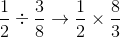You then treat the problem like any other multiplication problem and multiply straight across.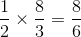Since the numerator is larger than the denominator, we know our answer will be greater than 1.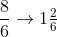6 divides into 8 one time, with 2 remaining. The 2 remains on top of the 6 as the numerator. This answer can still be reduced; however, as 2 and 6 are both evenly divisible by 2.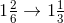Your final answer is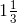### Example Question #1 : How To Multiply And Divide Fractions

One Australian dollar is equal to about 86 cents. To the nearest whole, for how many Australian dollars can a tourist to Australia expect to exchange $600 in American money? Possible Answers: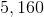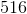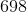Correct answer:Explanation: One Australian dollar is equivalent to 86 cents, or$0.86, in American money, so divide $600 dollars by this conversion factor.$600 is equivalent to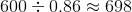Australian dollars.